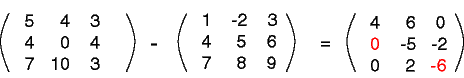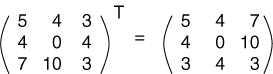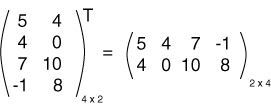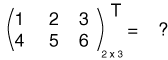Transpose

The transpose of a matrix is a new matrix whose rows are the columns of the original. (This makes the columns of the new matrix the rows of the original). Here is a matrix and its transpose:The superscript "T" means "transpose".

Another way to look at the transpose is that the element at row r column c in the original is placed at row c column r of the transpose. The element arc of the original matrix becomes element acr in the transposed matrix.

Usually we will work with square matrices, and it is usually square matrices that will be transposed. However, non-square matrices can be transposed, as well:QUESTION 14:

What is: